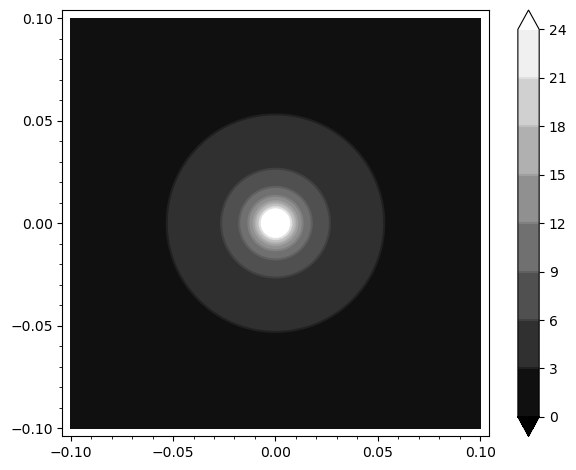# Contour Plot of 1/(2*pi*sqrt(x^2 + y^2)), why is it square? How to fix it?

I plotted the contour plot of a function 1/(2*pi*sqrt(x^2 + y^2)).

The code is below:

contour_plot(1/2/(pi*sqrt(x^2 + y^2)), (x, -0.1, 0.1), (y, -0.1, 0.1),
plot_points=20, colorbar=True)


The output result is not round, but square! Why?

How to fix it?

edit retag close merge delete

Sort by » oldest newest most votedThe plot_points argument affects the precision with which the plot is made; setting it low enough causes artifacts like circles appearing as squares. To get the image with those contours with more precision, you can omit the plot_points argument (or increase the value), and just pass the appropriate contours argument:

contour_plot(1/2/(pi*sqrt(x^2 + y^2)), (x, -0.1, 0.1), (y, -0.1, 0.1), contours=range(0,25,3), colorbar=True)more